Disclosure: This post may contain affiliate links, meaning when you click the links and make a purchase, we receive a commission.

# Using Excel to Round to Nearest 1000 (7 Easy Ways)

Sometimes it is often required to round numbers to its nearest hundreds or thousands. In this article, we will see how Excel round to nearest 1000. There are many formulas for doing that. Besides using different formulas, we will also see how Custom number formatting can be used to round numbers to its nearest 1000.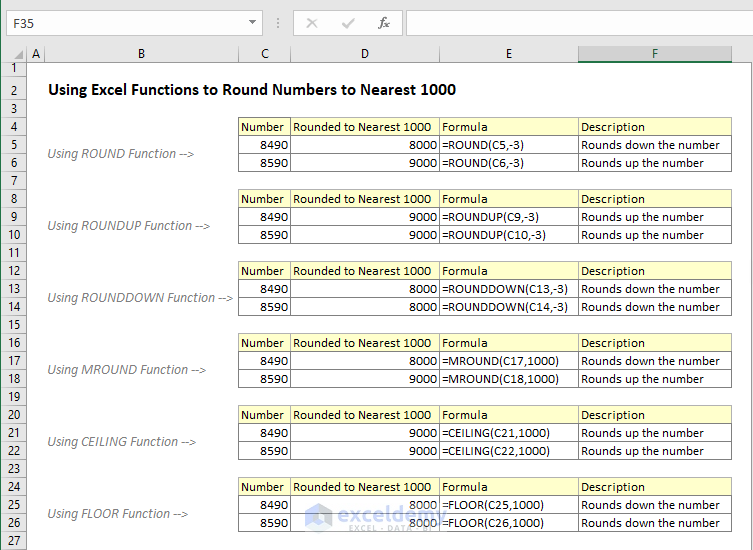Using Excel Functions to Round Numbers to Nearest 1000

## Round to Nearest 1000 using the ROUND Function

The best way to round numbers is to use the Round function. The syntax of the Round function is,

=ROUND( number, num_digits).

Here the argument number is the desired number which you want to round and num_digit is the number to which the desired number should be rounded up/down. If num_digits is greater than zero than the desired number will be rounded up to a specific number of decimal places counting from the right side of the decimal point. Similarly, if the num_digits is less than zero than the desired number will be rounded down. If num_digits=0 than the number will be rounded up to its nearest integer number.

Let`s insert some numbers in the worksheet to see how this ROUND function works. The number can be rounded up or rounded down depending on the value of the number. We will see two formulas with the ROUND function which will give us the same result.

The formulas that we will be using are,

• =ROUND (Cell, -3)
• =ROUND(Cell/1000,0)*1000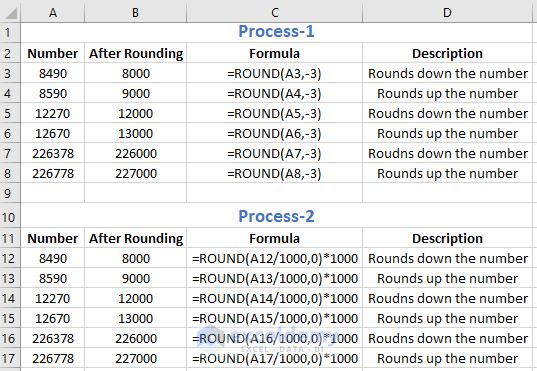From the above picture, it can be seen that with two different formulas give the same rounding result for the same number. Here we are working to round the number to its nearest 1000. For each of the numbers when the hundred place digit is greater or equal to number 5 the formula will round up the result. If the hundred place digit is less than 5 than the formula will round down the number.

## Excel Roundup to Nearest 1000

To round up any number to its nearest 1000 you can use the ROUNDUP function where the num_digit should be set as -3. So, the formula in this will be, =ROUNDUP (Cell, -3).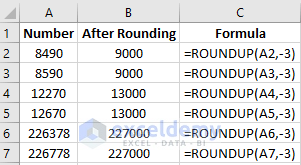## Excel Round Down to Nearest 1000

For rounding down a number to its nearest 1000 you need to use the ROUNDDOWN function. The argument, num_digit should be set as -3 again. So, the formula becomes, =ROUNDDOWN (Cell, -3).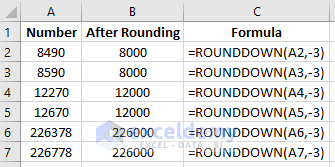## Excel Round to Nearest 1000 using the MROUND function

Another useful function that can be used to round number is the MROUND function. The syntax of the MROUND function is =MROUND (number, multiple). Here the number is the desired number which is rounded up/down. The multiple arguments are used here to round up or down the number to the nearest multiple of this value.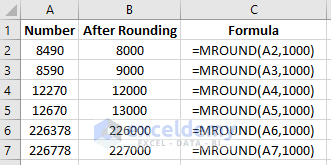In the above picture, we can see, the 2nd argument is set to 1000. So, any number will be rounded up/down to the nearest multiple of this value.

## Round Down Numbers to Nearest 1000 using the FLOOR Function

The FLOOR Function can be used to round down a number. Here we will round down different numbers to its nearest 1000. We will use different positive and negative numbers to see how the formula works.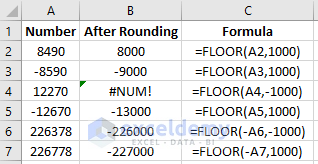From the above formula we can see that when we use a negative number in the 2nd argument which is the multiple to which you want to round, the 1st argument needs to be negative too. Otherwise, it will give a #NUM error.

Note: While rounding down a number to its nearest 1000 using the FLOOR function, the hundred place digit in a number doesn’t play any role like it does in the ROUND function.

## Excel Roundup to Nearest 1000 Using Ceiling Function

While the FLOOR function rounds down a number the ceiling function is used to round up a number. We can round up a number to its nearest 1000 using the CEILING function.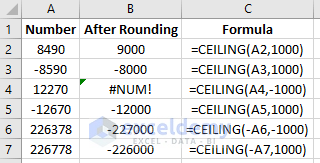Just like the FLOOR function. In the CEILING function when you use a negative number in the 2nd argument your first argument should also be negative otherwise it will give us a #NUM error.

Note: While rounding up a number to its nearest 1000 using the CEILING function, the hundred place digit in a number doesn’t play any role like it does in the ROUND function.

## Rounding a Number to its Nearest 1000 Using Custom Number Formatting

By using the custom number formatting, we can round up/down a number to its nearest 1000 value. Suppose you want to round the number 8490 to its nearest 1000. The nearest 1000 of 8490 is 8000. Using the custom number format, we can write this rounded number as 8k. The nearest thousand number of –8590 is -9000 which can be written as 9K using the custom number formatting. For doing this custom number formatting follow the steps below.

• First, select the cells where you want to apply the formatting. Here we select the cells B2: B7.
• Now after selecting them click the right button of the mouse to select the format cells option.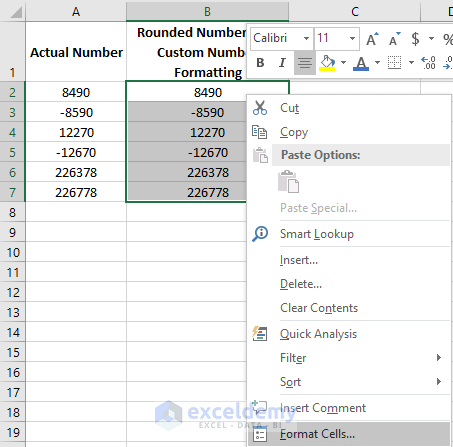• In the format cells dialogue box select Custom, and in the Type section write #,##0, K and press OK.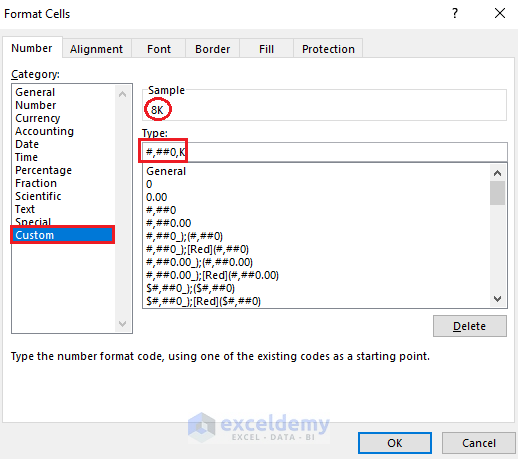• After doing this you will get to see the below result in your worksheet.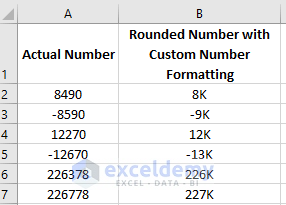## Rounding to the Nearest Thousand Worksheet

Rounding to the Nearest Thousand in Excel

## Conclusion

In this article, we saw different processes of rounding a number to its nearest 1000. We saw different formulas along with custom number formatting. Among all the Formulas, we can say using the ROUND or MROUND function is a good choice for rounding the number to its nearest thousands as we can both round up and round down the numbers with this single formula.

Hope you will like this article. Stay tuned for more useful articles and don’t forget to comment below if you face any difficulties.

## Related Articles

Hello!

Welcome to my Profile. Here I will be posting articles related to Microsoft Excel. I have completed my BSc in Electrical and Electronic Engineering from American International University-Bangladesh. I am a diligent, goal-oriented engineer with an immense thirst for knowledge and attitude to grow continuously. Continuous improvement and life-long learning is my motto.

1 Comment
1. ReplyN R RAVINDRA June 5, 2020 at 8:13 PM

is there a video on this, if not, pl make a video so that it can be understood very easily.

it is difficult to read and understand. If the same is explained in a video it can be understood very easily.